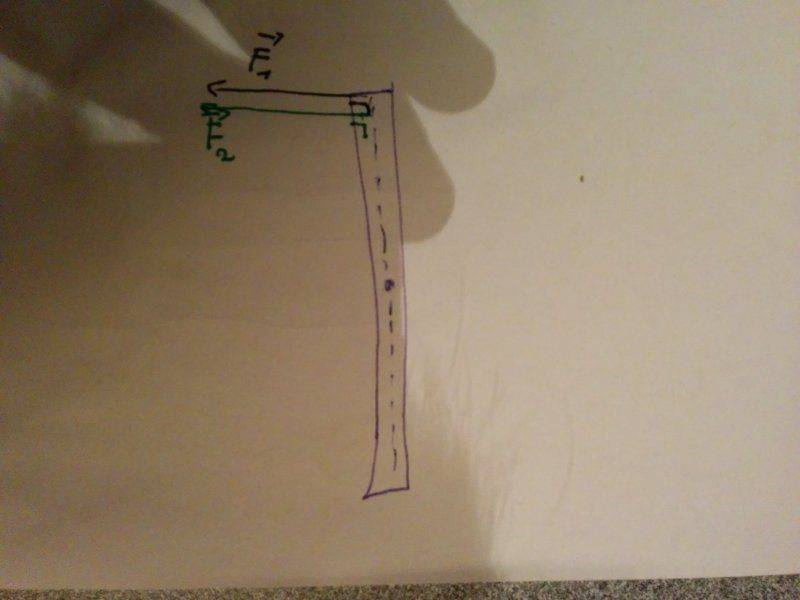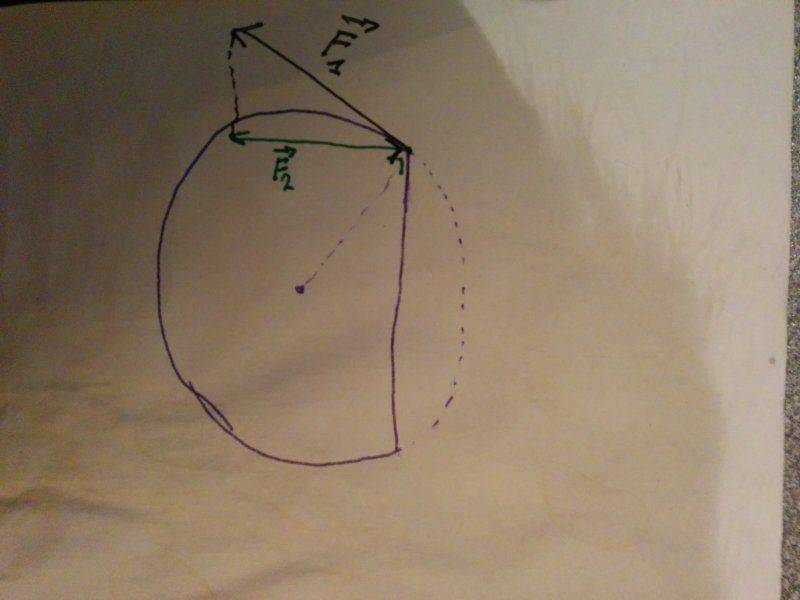# Most efficient torque transfer

I'm wondering what the most efficient way to transfer torque is. I'm not talking about which shape can handle the most torque but rather how can I transfer 100% of the torque. Lets take screw bits for example. It seems to me that the simple slotted or philips head transfer 100% of the torque because the force of rotation generated by the torque is applied completely perpendicular to the surface and hence all the force (and torque) is transfered. Compare this to for example an allen or a torx where the force is not perpendicular and hence some force gets waisted. Is this correct?

If yes I have the following issue. I have the following two metal surfaces sticking out as in the images attached which I am trying to rotate (with e.g. a screwdriver). In the circular one, the torque (generated by the screwdriver) generates the largest force in the F1 direction. However, that's not possible given the shape of it so it can only apply force in the F2 direction, which is the projection of F1 onto the perpendicular direction of the flat surface. On the flat surface sticking out, the torque generates maximum force perpendicular to the surface and hence this would transfer 100% of the torque. (the exact same wound he true for any flat surfaces. such as a philips.) Am I correct here? Thank you very much for your time!Actually, the more I think about it the more I'm coming to the conclusion that "if there is no slip, 100% of torque is transfered", the internal forces just vary with the different shapes but ultimately all torque is transfered. Anyway, some help is always welcome =).

CWatters
Homework Helper
Gold Member
Correct.

Consider also what happens if the screw it turning at a constant angular velocity (which could be zero). If the screw doesn't accelerate then the forces acting on it sum to zero. eg At constant angular velocity the torque applied by the driver exactly matches that due to friction between the screw and the material it's in.

Thanks for the reply! But lets say that there is a little slip due to the 2 parts not fitting perfectly, and maybe some material deformation (the object I'm using to turn the heads is made out of ABS plastic). Could the shape of the knob matter in this situation? That is, would it be easier for me to turn the flat head rather than the rounded head (and would it be noticeable?) or is the reply again, the forces are just better distributed when using the flat head but the torque applied is identical?

CWatters